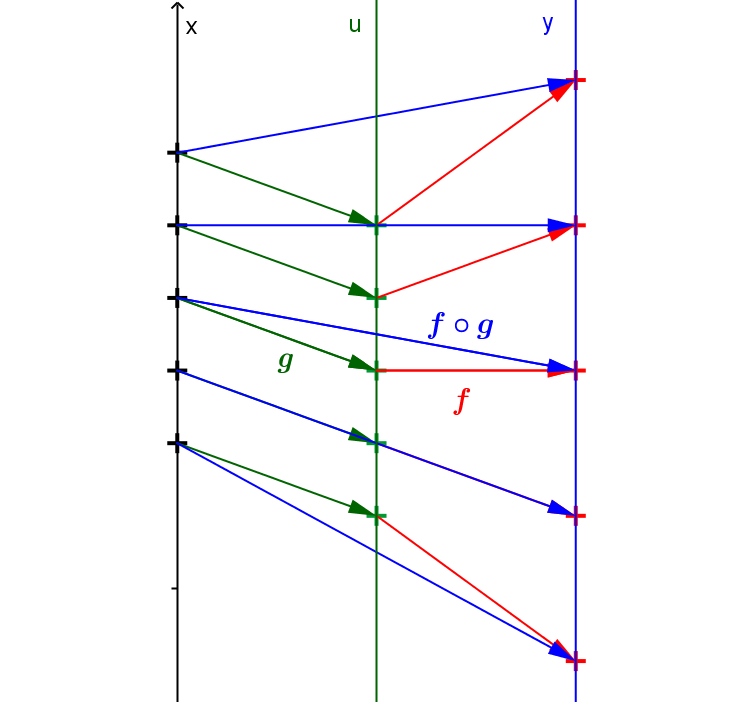Definition: Suppose $f$ and $g$ are functions with  $g: D_g \rightarrow R$ and $f: D_f \rightarrow R$ where for ever $x \in D_g, g(x) \in D_f$. The composition of $f$ with $g$, denoted $f \circ g$, is the function defined on $D_g$ by $(f \circ g)(x) = f(g(x))$ for all $x \in D_g$.Mapping Diagram: $f \circ g : x \rightarrow g(x) \rightarrow f(g(x))$